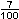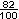# Writing Percents as DecimalsProblem: What is 35 percent of one dollar?

We know from the previous lesson that .35 = 35%. The word "of" means multiply. So we get the following:

35% x \$1.00 = .35 x \$1.00

since \$1.00 = 1, we get .35 x \$1.00 = .35 x 1 = .35

35% of \$1.00 is \$.35

Solution: 35% of one dollar is \$.35, or 35 cents.

The solution to the above problem is not surprising, since percents, dollars and cents are all based on the number 100. To convert a percent to a decimal, first remove the percent symbol, then move the decimal point two places to the left. Look at the Example 1 below.

Example 1: Write each percent as a decimal:     18%,    7%,    82%,    55%

 Solution Percent Decimal 18% .18 07% .07 82% .82 55% .55

In Example 1, it was easy to convert each percent to a decimal since each percent had two digits. (The exception to this was 7% for which we needed to add in a zero in order to make the conversion.) Thus, we need a procedure to follow for consistency.

To write a percent as a decimal, follow these steps:

1. Remove the percent symbol.
2. Move the decimal point two places to the left, adding in zeros as needed.

#### Why do we move the decimal point 2 places to the left?

Remember that percent means parts per hundred, so 18% =From your knowledge of decimal place value, you know that= 0.18  (eighteen hundredths).

Thus, 18% must also equal 0.18.

Let's take another look at Example 1, this time including the fractional equivalents of each percent.

Example 1: Write each percent as a decimal:     18%,    7%,    82%,    55%

 Solution Percent Fraction Decimal 18%.18 07%.07 82%.82 55%.55

Let's look at another example of writing percents as decimals.

Example 2: Write each percent as a decimal:     12.5%,    89.19%,    39.2%,    71.935%

 Solution Percent Decimal 12.5% .12500 89.19% .89190 39.2% .39200 71.935% .71935

Summary: To write a percent as a decimal, remove the percent symbol, then move the decimal point two places to the left, adding in zeros as needed.

### Exercises

Directions: Read each question below. Select your answer by clicking on its button. Immediate feedback is provided in the RESULTS BOX. If you make a mistake, choose a different button.

 1.  Which of the following is equal to 64%? .064       .64       6.74       None of the above.       RESULTS BOX:
 2.  Which of the following is equal to 7.27%? 7.27       .727       .0727       None of the above.       RESULTS BOX:
 3.  Which of the following is equal to 91.4763%? .914763       9.14673       91.4763       None of the above.       RESULTS BOX:
 4.  Which number has the greatest value? 19.72%       1.972       \$19.72       20 cents       RESULTS BOX:
 5.  Which number has the least value? 1.039%       1.39       \$1.39       139 cents       RESULTS BOX: